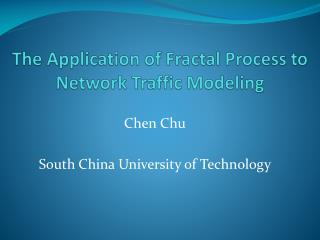DownloadDownload PresentationThe Application of Fractal Process to Network Traffic Modeling

# The Application of Fractal Process to Network Traffic Modeling

Télécharger la présentation## The Application of Fractal Process to Network Traffic Modeling

- - - - - - - - - - - - - - - - - - - - - - - - - - - E N D - - - - - - - - - - - - - - - - - - - - - - - - - - -
##### Presentation Transcript

1. The Application of Fractal Process to Network Traffic Modeling ChenChu SouthChinaUniversityofTechnology

2. 1.Self-SimilarprocessandMulti-fractalprocess • Thereare3differentdefinitionsforself-similarprocess and 2 different definitions of multi-fractal process. • Definition1: Acontinuous-timeprocessY(t)isself-similarifitsatisfies: Y(t)=a-HY(at)foranya>0,0≤H<1 The equality means finite-dimensional distributions. This process can not be stationary, but it is typically assumed to have stationary increments. Fractional Brownian Motion(FBM) is such a process. The stationary increment process of FBM is FGN. • DefinitionI of multi-fractal: A multi-fractal process Y(t) satisfies: Y(t) = a-H(t)Y(at) foranya>0,0≤H(t)<1 Multi-fractional Brownian Motion is such a process. It’s neither a stationary process nor a stationary increment process.

3. 1.Self-SimilarprocessandMulti-fractalprocess • Definition2: Awide-sense stationary sequence X(i). For each m = 1, 2, 3,..., Let Xk(m) ＝1/m(Xkm-m+1＋ …＋Xkm)，k＝1, 2, 3, … The process X is called self-similar process if it satisfies the following conditions: 1) Theautocorrelationfunctionr(k) is a slowly varying function. 2) r (m) (k) = r(k) If the condition 2) is satisfied for all m, then X is called exactly self-similar. If the condition 2) is satisfied only for m becomes infinite, then X is asymptotically self-similar. FGN is exactly self-similar process. FARIMA is asymptotically self-similar process.

4. 1.Self-SimilarprocessandMulti-fractalprocess • Definition3 of Self-Similar process: For a time series X and its aggregated process X(m), Let μ(m) (q) = E | X(m) |q If X is self-similar, then μ(m) (q) is proportional to m , so we have the following formulas: 1): log μ(m) (q) = β(q) log m + C(q) 2): β(q) = q(H-1) • Definition 2 of multi-fractal process: β(q) is not linear with respect to q. In other words, for different q we get different H.

5. 1.Self-SimilarprocessandMulti-fractalprocess

6. 1.Self-SimilarprocessandMulti-fractalprocess

7. 2.TheCharacteristicsofNetworkTraffic • 1):Self-similarityorscalingphenomena.However,theself-similarityexistsindifferentscalesfordifferentnetwork. BC-89Aug FrameTraffic: Thescalingphenomenaexistsfrom10msto100s.

8. 2.TheCharacteristicsofNetworkTraffic • MAWIIPv6WIDEbackbone. • Thescalingphenomenaexistduring100ms~100s.Fortimescalelessthan100ms,thereisnoself-similarexist.

9. 2.TheCharacteristicsofNetworkTraffic • 2):Themarginaldistributionofnetworktrafficisnotnormal.Butas the time scale increase, it becomes normal.

10. 2.TheCharacteristicsofNetworkTraffic • 3):Thelong-dependence of the traffic.

11. 2.TheCharacteristicsofNetworkTraffic

12. 2.TheCharacteristicsofNetworkTraffic • Conclusion: • Larger scales (time scale larger than 10ms or even 100ms) self-similarity long-dependence nearly normaldistribution. • Small scales (time scale less than 100ms) any distribution (usually lognormal or heavy-tail) not self-similar short-dependent

13. 2.TheCharacteristicsofNetworkTraffic • Models for network traffic For large scales: FGN is a suitable model. For small scales: 1) generate non-normal FARIMA time series with length n; 2) divide the FARIMA time series into k different series, permute each of these series.

14. 3.TheEstimationofHurstParameter • The estimation of the Hurst parameter has a close relationship with the marginal distribution of the time series. Estimationof Hwith100independentFGN(10^5long)

15. 3.TheEstimationofHurstParameter Estimationof Hwith50independentFARIMA(10^5long) (marginal distribution is heavy-tailed with tail parameter alpha = 1.8)

16. 3.TheEstimationofHurstParameter Estimationof Hwith50independentFARIMA(10^5long) (marginal distribution is heavy-tailed with tail parameter alpha = 1.6)

17. 3.TheEstimationofHurstParameter Some of the method does not suitable for the non-normal self-similar time series. Absolute moment method and higuchi’s method often overestimate the H for non-normal self-similar time series. The different variance method has large estimated variance.